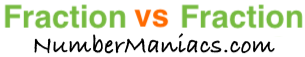1/2 vs 5/8People compare 1/2 vs 5/8 drywall, 1/2 vs 5/8 pipe, 1/2 vs 5/8 cup, 1/2 vs 5/8 ton, 1/2 vs 5/8 drill bit, 1/2 vs 5/8 poker, 1/2 vs 5/8 sump pump, 1/2 vs 5/8 garden hose, and many other items.

Is 1/2 greater than 5/8? Is 1/2 less than 5/8? What is the difference between 1/2 and 5/8? These and other questions are answered below so you can get a better perspective of 1/2 vs 5/8.

1/2 vs 5/8 Fractional Comparisons
1/2 is less than 5/8
The difference between 1/2 and 5/8 is 1/8
1/2 is 1/8 less than 5/8

1/2 vs 5/8 Decimal Comparisons
1/2 vs 5/8 as a decimal is 0.5 vs 0.625
The difference between 1/2 and 5/8 is 0.125
1/2 is 0.125 less than 5/8

1/2 vs 5/8 Percent Comparisons
1/2 vs 5/8 as a percent is 50% vs 62.5%
1/2 is 20 percent less than 5/8

Fraction vs Fraction
Submit another set of fractions that we can compare for you!

/
vs
/

The math behind the numbers
Calculations on this page are rounded to the nearest thousandth if necessary. Below is the math we used to analyze 1/2 vs 5/8:

To compare the size of 1/2 vs 5/8, we simply subtracted 5/8 from 1/2:

1/2 - 5/8 = |1/8|

To find 1/2 vs 5/8 as a decimal, we divided the numerator by the denominator for each fraction.

1 ÷ 2 = 0.5
5 ÷ 8 = 0.625

To find 1/2 vs 5/8 as a percent, we divided the numerator by the denominator and then multiplied the quotient by 100 for each fraction.

(1 ÷ 2) x 100 = 50%
(5 ÷ 8) x 100 = 62.5%

We found that 1/2 is 20 percent less than 5/8 by dividing the difference between the two fractions by the second fraction and then multiplied it by 100:

((1/8)/(5/8))×100 = 20%

That's it folks. We hope this page gave you a better understanding of 1/2 vs 5/8 with our explanations and conclusions.

1/2 vs 5/9
Here are the next two fractions we have compared and analyzed.